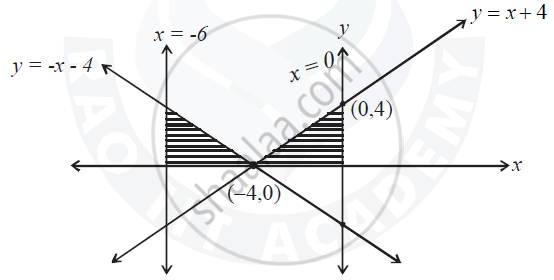ISC (Commerce) Class 12CISCE
Share

# Sketch the Graph of Y = |X + 4|. Using Integration, Find the Area of the Region Bounded by the Curve Y = |X + 4| and X = –6 and X = 0. - ISC (Commerce) Class 12 - Mathematics

ConceptArea of the Region Bounded by a Curve and a Line

#### Question

Sketch the graph of y = |x + 4|. Using integration, find the area of the region bounded by the curve y = |x + 4| and x = –6 and x = 0.

#### Solution

y = x + 4, if x > 4 and y = -(x+4), if x < 4For y = x + 4

when x = 0, y= 4

and when y = 0, x = -4

Point are

∴ (0,4) and (-4,0)

For y = -x - 4

when x = 0,  y = -4

when y = 0, x= -4

∴ Point are

(0, -4) and (-4,0)

∴ Required area

= int_(-6)^(-4)-(x+4) dx + int_(-4)^0 (x + 4) dx

= -[x^2/2 + 4x]_(-6)^(-4) + [x^2/2 + 4x]_(-4)^0

= [(-4)^2/4 + 4(-4) - [(-6)^2/2 + 4(-6)]] + [0 + 0[(-4)^2/2 + 4(-4)]]

= -[16/2 - 16 -[36/2 - 24]] + [-(16/2 - 16)]

= -[-8 + 6] + 

2 + 8 = 10 sq. unit

Is there an error in this question or solution?

#### Video TutorialsVIEW ALL 

Solution Sketch the Graph of Y = |X + 4|. Using Integration, Find the Area of the Region Bounded by the Curve Y = |X + 4| and X = –6 and X = 0. Concept: Area of the Region Bounded by a Curve and a Line.
S